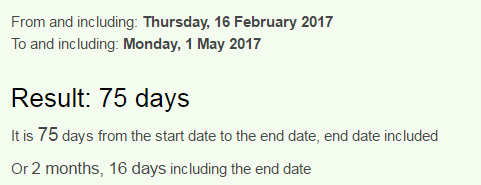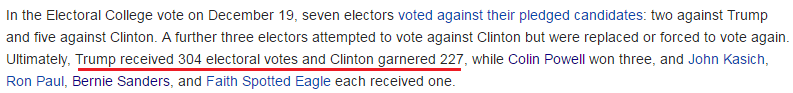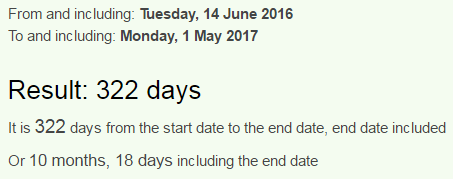## Cipher## Feb 16, 2017

### 2/16 Time Cover 187 Trump assassination

Once again we see assassination codes for Trump... 10 days after he won the election I got a hint from a TV show about his assassination and it seems like a lot of the stuff is pointing to May Day in 2017... I will quickly explain how this connects to my prediction since I already covered a lot of the May 1st stuff in the last few monthsNothing to see here = 5+6+2+8+9+5+7 + 2+6 + 1+5+5 + 8+5+9+5 = 88 (Reduced)
Trump = 20+18+21+13+16 = 88 (Ordinal)
Nothing to see here = 4+3+7+1+9+4+2 + 7+3 + 8+4+4 + 1+4+9+4 = 74 (Reverse Reduced)
From 2/16 to May 1st is 74 days if we don't count the last day, but counting it gives us 2 months and 16 days...Nothing to see here = 14+15+20+8+9+14+7 + 20+15 + 19+5+5 + 8+5+18+5 = 187 (Ordinal)
Nothing to see here except the big homicide code. Note the sum of divisors on 187... Homicide Code = 93 (Ordinal)216 (6x6x6)
May First = 78+6+150 + 36+54+108+114+120 = 666 (Sumerian)
May 1st also comes 135 days after Saturnalia in 2016, 93 in octal system is 135(+531) = 666
Saturnalia starts on "December Seventeenth" = 93 - S exception
May Day = 14+26+2 + 23+26+2 = 93 (Reverse Ordinal)Trump got 304 and Hillary got 227 electoral votes....... 304+227 = 531(+135) = 666
Super Bowl 51 came 2 months and 27 days after Trump won the election vote on 11/9/2016, and now from Super Bowl to May first there is 2 months and 27 days

This is a screenshot from Zach's post http://freetofindtruth.blogspot.rs/2017/02/47-74-88-97-187-1122-nothing-to-see.htmlThis year Saturnalia comes 187 days after Trump's birthday and it's interesting how Pope Francis is celebrating his birthday on Saturnalia, if Trump "dies" on 5/1 it will be 44 days before his 71st birthdayPope Francis = 60+50+60+5 + 6+80+1+40+3+9+90 = 404 (Jewish), he appeared on Super Bowl 51 on a date with 44 numerology
Saturnalia = 10+1+2+3+9+5+1+3+9+1 = 44 (S Exception)President = 47 - TIME = 20+9+13+5 = 47 (Ordinal), Trump = 7+9+6+14+11 = 47 (Reverse Ordinal), Trump = 100+80+200+30+60 = 470 (Jewish), Republican = 9+5+7+3+2+3+9+3+1+5 = 47 (Reduced)

2/16 leaves 318 days in the year which connects to Lucifer episode that came out on 11/14 (normally 318th day in the year), where Lucifer talks about Trump dying soon, note that the TV show went on a break until the first of May after releasing an episode named "A good day to die"Candy Morningstar = 6+8+4+5+2 + 5+3+9+4+9+4+2+8+7+8+9 = 93 (Reverse Reduced)
May Day = 14+26+2 + 23+26+2 = 93 (Reverse Ordinal)
The episode that came out on 11/14/2016 is called "Trip to stabby town" and Lucifer said Trump in the 44th second of the 38th minute (Kill = 44, Execution = 44, Death = 38, Killing = 38)Trump appears on TIME cover 3 months and 3 days after this episode came out, but there is interesting connection to his previous appearance on TIME which was 3 weeks and 3 days after this episodeThe last time Trump appeared on the cover of Time magazine was on Pearl Harbor day on 12/7/2016, and the date has absolutely matching date numerology with February 16th that comes 71/72 days later
12/7/2016 - 1+2+7 + 2+0+1+6 = 19    ---------  2/16/2017 - 2+1+6 + 2+0+1+7 = 19
12/7/2016 - (12) + (7) + 2+0+1+6 = 28  --------- 2/16/2017 - (2) + (16) + 2+0+1+7 = 28 (This is coming 28 days after Trump entered office)
12/7/2016 - (12) + (7) + (20) + (16) = 55  --------- 2/16/2017 - (2) + (16) + (20) + (17) = 55
12/7/16 -  (12) + (7) + (16) = 35  --------- 2/16/17 - (2) + (16) + (17) = 35Note that this is a span of 71 days and Trump could "die" 44 days before turning 71If we include the last day this Time cover is coming 72 days from Pearl Harbor day
Pearl Harbor day = 7+5+1+9+3 + 8+1+9+2+6+9 + 4+1+7 = 72 (Reduced)
Pearl Harbor day = 16+5+1+18+12 + 8+1+18+2+15+18 + 4+1+25 = 144 (Ordinal)
TIME = 100+9+30+5 = 144 (Jewish)
216 written out also connects to 144
Two one six = 20+23+15 + 15+14+5 + 19+9+24 = 144 (Ordinal)
Trump is also the 44th man to be the president

If he dies on May 1st it will also be 322 days from his last birthday (322 is the number of Skull and Bones and the reflection is "The Synagogue of Satan" = 223)May First = 5+8+2 + 3+9+9+8+7 = 51 (Reverse Reduced)
First of May = 6+9+9+1+2 + 6+6 + 4+1+7 = 51 (Reduced)
First of May = 21+18+9+8+7 + 12+21 + 14+26+2 = 138 (Reverse Ordinal)
Donald Trump = 4+15+14+1+12+4 + 20+18+21+13+16 = 138 (Ordinal)
We just saw Super Bowl 51... and I wouldn't be surprised for a second if they really have the date set for 5/1/2017.. There's so much crazy coding all around Trump.... I have at least 5 posts from the last 2 months covering a lot of Trump assassination stuff

1.Had pop'ed this on google, but few things that seem to connect ...
Trump is Mr. Time. 'Mr. Time' = 33. 'Dr. Time' = 33.
With 'Trump' = 88 & the headline ... If you sum the first 33 prime numbers, they = 1988 (88).
In the Tarot Deck, the first Major Arcana or 'Trump' card, is 'The Magician'. 'The Magician' = 213. 'Tarot Cards' = 119.
'Saturn The Keeper Of Time' = 119 in reduction.
The Magician is depicted with the infinity symbol above his head, the sideways '8'. 'Infinity' = 106. 'Prophecy' = 106. 'Skull And Cross-bones' = 106. 'Three Hundred Twenty Two' = 106.
213 can connect to Skull & Bones no. 322. ...
Prime factorization for the no. 322 = 2 x 7 x 23.
'Two multiplied by Seven multiplied by Twenty Three' = 213. The no. 322 has a total of '8' divisors ...
Infinity = an 8, rotated 90 degree's almost.
Divisors of 322 summed = 576 Sumerian. 'Freemason' = 576. 'Three Two Two' = 55. ( Satan / Lightning )
And the date 2/16. (216). ... Time(144) + 216 = 360 ( The circle or The Sun ) ... 216 years = 3 degree's of the Great Processional of the Zodiac. 72 years = 1 degree.

Class stuff man, All the best, Dar.

1.Beautiful finds man, You are right on point with 144+216 stuff, something is coming full circle for them very soon, you mention 322, if they "assassinate" him on May 1st it will be 322nd day after his last birthday... which is pretty wild as well... Trump will be remembered as the most Saturnian president in a few years haha
Three hundred sixty = 227, and there is plenty of 227 stuff coming up with Trump and this election

2.Excellent work!